HomePuzzle ContestSingle Shift PuzzleFlip Flat RubikDouble Shift PuzzleRotate 3x3 PuzzleRotate 5x5 PuzzleRotate 7x7 PuzzleNine Faces RubikSix Faces Rubik24 Faces Magic Cube4D Rubik CubeQuotes By AuthorQuotes By SubjectQuote Of The DayDetective MysteryReversiLogic TestMath TestWord Of The DayUnsolved ProblemsHall of FameContact UsLoginSign UpSign OutMy AccountChange PasswordAbout UsContributorsLink To UsPrivacy PolicyTerms of ServiceSite Map

## Flat Rubik - 4D Rubik Cube Puzzle Rules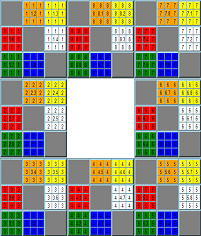Flat 4D Rubik Cube Puzzle is a flat equivalent of a four-dimensional variation of the Rubik Cube puzzle. A four-dimensional space (4D) is an abstract mathematical generalization of a three-dimensional space.
4D Rubik Cube consists of 8 cells. Each cell represents the original three-dimensional (3D) Rubik Cube. Cell #1 is presented on Figure 1. As the original 3D Rubik Cube, it consists of 6 faces. Each face has a different color.Figure 1
A vector with four elements (xyzv) can be used to represent a position of a 4D cube in four-dimensional space. See more details in Appendix 1.

4D Cube has 24 faces. Each face belongs to two cells (3D cubes). For example, the face 1.2 belongs to the cell #1. It has coordinates X=0, Y=0 (See Appendix 1). Face 2.1 belongs to the cell #2 and has coordinates X=0, Y=0. Since the face 2.1 has the same coordinates as the face 1.2, it is just a mirror of the same face.

The central square of each face displays two digits separated by dot. The first digit is the cell number. The second digit shows the cell number for the mirror face. For example, face 1.2 belongs to the cell #1, and its mirror (2.1) face belongs to the cell #2. In this example the mirror face 2.1 belongs to the cell #2, and its mirror face (1.2) belongs to the cell #1. All squares except the central squares show only the cell number.

You can rotate the cell by clicking on any of the central squares. There are four rotating options: Rotate One Layer Clockwise, Rotate One Layer Counterclockwise, Rotate 3D Cube (the whole cell) Clockwise, and Rotate 3D Cube (the whole cell) Counterclockwise. For the puzzle to be solved, all squares should return to their original places.

Rotate One Layer example
For example, if you select the ‘Rotate One Layer Clockwise' option and click on “1.2” square, the following elements of the 4D Cube will be moved (See Appendix 2 for details):

• One layer attached to the face 1.2 in the cell #1 rotates clockwise. One layer rotation is similar to the rotation of the Six Faces Flat Rubik Puzzle (See Appendix 3)
• One layer attached to the face 2.1 in the cell #2 rotates counterclockwise. One layer rotation is similar to the rotation of the Six Faces Flat Rubik Puzzle (See Appendix 3)
• The edge AB in the cell #8 moves to the cell #3 and replaces the edge DA.
• The edge BC in the cell #7 moves to the cell #8 and replaces the edge AB.
• The edge CD in the cell #4 moves to the cell #7 and replaces the edge BC.
• The edge DA in the cell #3 moves to the cell #4 and replaces the edge CD.

The results of the rotation are shown on Figure 2.
Figure 2
 Cell #1(X=0)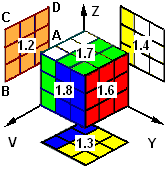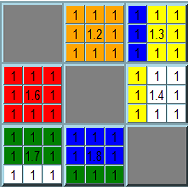Cell #2(Y=0)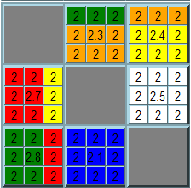Cell #3(Z=0)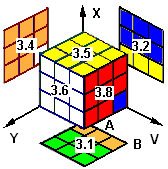Cell #4(V=0)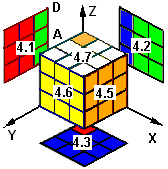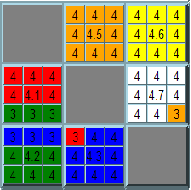Cell #7(Z=1)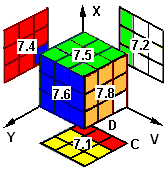Cell #8(V=1)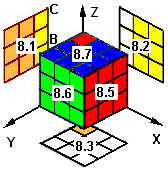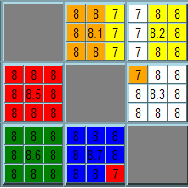Rotate 3D Cube (the whole cell) example
If you select the ‘Rotate Cell Clockwise' option and click on “1.2” square, the following elements of the 4D Cube will be moved:

• The whole cell #1 rotates clockwise including the following elements: the face 1.2 rotates clockwise; the face 1.6 rotates counterclockwise; the face 1.3 moves to 1.4; face 1.4 moves to 1.7; the face 1.7 moves to 1.8; and the face 1.8 moves to 1.3.
• One layer attached to the face 2.1 in cell #2 rotates counterclockwise
• One layer attached to the face 6.1 in cell #6 rotates clockwise
• One layer attached to the face 3.1 in cell #3 moves to the layer 4.1 in cell #4
• One layer attached to the face 4.1 in cell #4 moves to the layer 7.1 in cell #7
• One layer attached to the face 7.1 in cell #7 moves to the layer 8.1 in cell #8
• One layer attached to the face 8.1 in cell #8 moves to the layer 3.1 in cell #3

The result of the rotation is shown on Figure 3.
Figure 3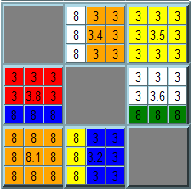Cell #1 Cell #2 Cell #3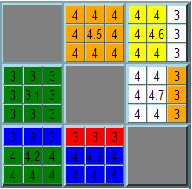Cell #4 Cell #7 Cell #8
Appendix 1

The Figure 4 below shows the relationship between 4D Rubik’s Cube faces, Flat Rubik faces, and four (xyzv) coordinates for each cell.
Figure 4
 Cell #1: X = 0 Y=0 =>1.2 Y=1=>1.6 Z=0=>1.3 Z=1=>1.7 V=0=>1.4 V=1=>1.8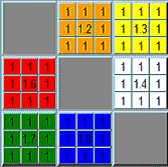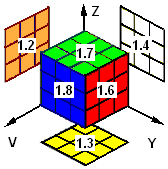Cell #2: Y = 0 X=0=>2.1 X=1=>2.5 Z=0=>2.3 Z=1=>2.7 V=0=>2.4 V=1=>2.8Cell #3: Z = 0 X=0=>3.1 X=1=>3.5 Y=0=>3.2 Y=1=>3.6 V=0=>3.4 V=1=>3.8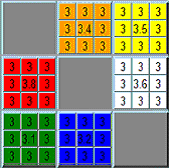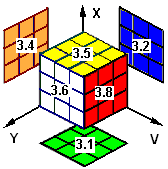Cell #4: V = 0 X=0=>4.1 X=1=>4.5 Y=0=>4.2 Y=1=>4.6 Z=0=>4.3 Z=1=>4.7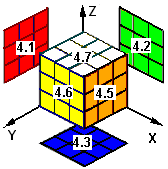Cell #5: X = 1 Y=0 =>5.2 Y=1=>5.6 Z=0=>5.3 Z=1=>5.7 V=0=>5.4 V=1=>5.8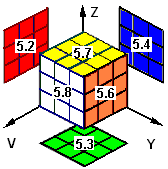Cell #6: Y = 1 X=0=>6.1 X=1=>6.5 Z=0=>6.3 Z=1=>6.7 V=0=>6.4 V=1=>6.8Cell #7: Z = 1 X=0=>7.1 X=1=>7.5 Y=0=>7.2 Y=1=>7.6 V=0=>7.4 V=1=>7.8Cell #8: V = 1 X=0=>8.1 X=1=>8.5 Y=0=>8.2 Y=1=>8.6 Z=0=>8.3 Z=1=>8.7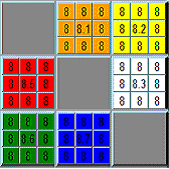Appendix 2

Each face has four edges and eight vertices. The vertices on the face 1.2 have the following coordinates: A(0,0,0,1), B(0,0,1,1), C(0,0,1,0), D(0,0,0,0). The vertices A, B, C, and D besides cell #1 belong to the cells #2, 3, 4, 7, and 8 (See the Figure 5).

If you select the ‘Rotate One Layer Clockwise' option and click on “1.2” square, the vertices will have the following coordinates: B(0,0,0,1), C(0,0,1,1), D(0,0,1,0), A(0,0,0,0) (See Figure 5 below).
Figure 5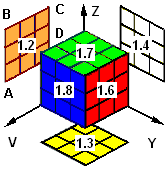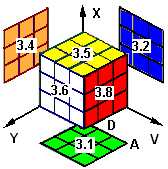Cell #1 (X=0) Cell #2 (Y=0) Cell #3 (Z=0)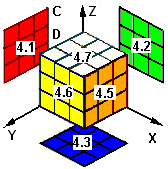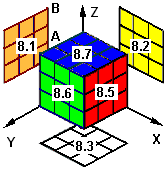Cell #4 (V=0) Cell #7 (Z=1) Cell #8 (V=1)
Appendix 3

Rotating one layer is similar to the rotation of the Six Faces Flat Rubik Puzzle. Figure 6 displays the one layer (attached to the face 1.2 in cell #1) rotation for the original Rubik Cube.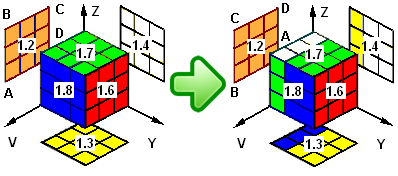Figure 6
Figure 7 displays the equivalent of one layer rotation for the Flat Rubik Puzzle. It shifts and rotates clockwise 12 elements around the selected face 1.2.Figure 7